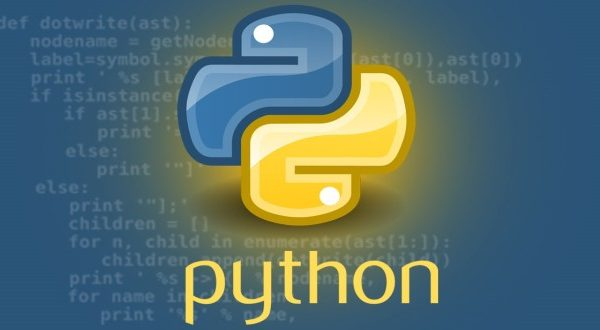# Kernel Density Estimation in Python Using Scikit-Learn### Introduction

This article is an introduction to kernel density estimation using Python’s machine learning library `scikit-learn`.

Kernel density estimation (KDE) is a non-parametric method for estimating the probability density function of a given random variable. It is also referred to by its traditional name, the Parzen-Rosenblatt Window method, after its discoverers.

Given a sample of independent, identically distributed (i.i.d) observations ((x_1,x_2,ldots,x_n)) of a random variable from an unknown source distribution, the kernel density estimate, is given by:

\$\$
p(x) = frac{1}{nh} Sigma_{j=1}^{n}K(frac{x-x_j}{h})
\$\$

where (K(a)) is the kernel function and (h) is the smoothing parameter, also called the bandwidth. Various kernels are discussed later in this article, but just to understand the math, let’s take a look at a simple example.

#### Example Computation

Suppose we have the sample points [-2,-1,0,1,2], with a linear kernel given by: (K(a)= 1-frac{|a|}{h}) and (h=10).